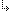- 4.45.2.41. -4. Standard Units4.45. Math - Extended Mathematics unit4.45.2. Math Unit Procedures and Functions4.45.2.41. LRotL function

## 4.45.2.41. LRotL function

Targets: MS-DOS, OS/2, Win32

Math Unit

Rotate bits to the left (LRotL) or right (LRotR).

Declaration:
```function LRotL(Value, Shift: DWORD): DWORD;
function LRotR(Value, Shift: DWORD): DWORD;```
Remarks:
The LRotL and LRotR functions rotate Value by Shift bits. LRotL rotates the value left. LRotR rotates the value right. Both functions “wrap” bits rotated off one end of Value to the other end.

- 4.45.2.41. -